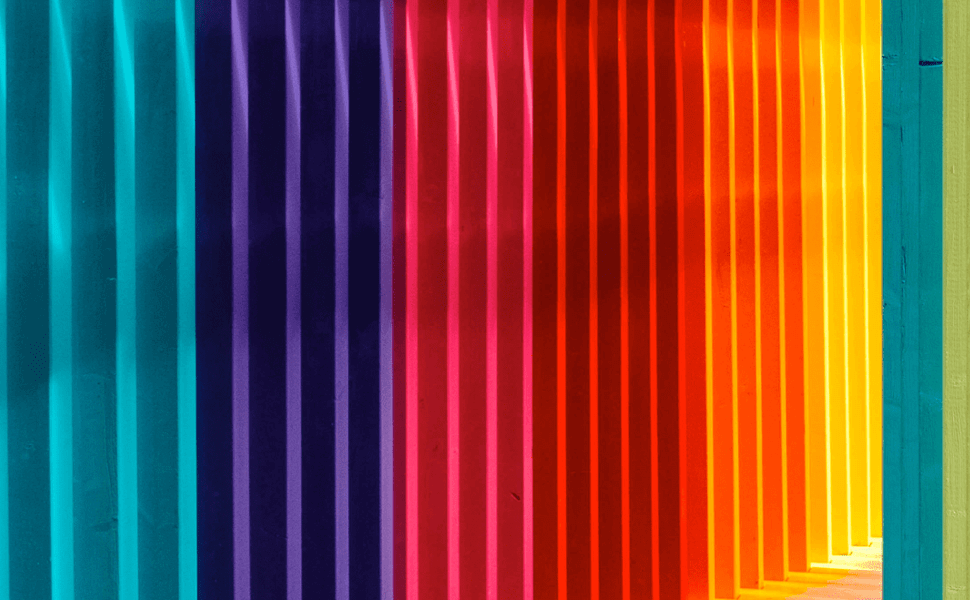# UIColor from HEX that Works!## TL;DR

A simple Swift extension to initialize `UIColor` from a hex string taking into consideration edge cases and hex representation variations

If you’re using an API to get colors to use them in your UIKit app, it is very likely that you’re getting them in hex format.

Representing colors with a hexadecimal number is the industry standard and widely used everywhere, which keeps me wondering why on earth doesn't Apple offer a built-in initializer to create a `UIColor` object from a hex value!

While many answers on Stack Overflow works just fine, they ignore the problem that the same color might be represented in many ways using hexadecimal format.

A quick search on Github or cocoapods.org will return many libraries to create `UIColor` from a hex string. Please don't add another dependency to your project just for this, it is just a few lines of code!

## Edge Cases

#### 1. Optional `#` Prefix

The hex string might -or might not- start with the `#` prefix, so `FF6347` and `#FF6347` are both valid representation for tomato color.

#### 2. Optional `0x` Prefix

The hex string might -or might not- start with the `0x` prefix, so `FF6347` and `0xFF6347` are both valid representation for tomato color.

#### 3. Representation Length

The standard length for a color representation in hex format is 6 (2 digits for each channel: red, green, and blue)

• `#000000`: black
• `#FFFFFF`: white
• `#FF0000`: red
• `#00FF00`: green
• `#0000ff`: blue

Other representations might add another two digits to the end to represent the alpha channel (transparency).

• `#FF000080` is the color red with 50% transparency - where 80 is the hex representation for 128 in decimal, (approximately 255/2).

Both above representations can be shorthanded to 3 and 4 digits respectively when each adjacent pair of digits are the same

• `#FF0000` becomes `#F00`
• `#AA00BBCC` becomes `#A0BC`

#### 4. Invalid Input

The string might be completely invalid

• `##FF0000`: contains more than # in prefix
• `AAFFNJ`: not a valid hex number
• `Lorem Ipsum`: normal string
• `#00FF00FF00FF`: completely valid hex number but does not have a valid length for a color representation

Here is a simple extension to `UIColor` that takes care of all above edge cases, the initializer is failable to avoid crashes when the hex string is invalid

swift

```extension UIColor { convenience init?(hex: String) { var hexString = hex if hexString.hasPrefix("#") { // Remove the '#' prefix if added. let start = hexString.index(hexString.startIndex, offsetBy: 1) hexString = String(hexString[start...]) } if hexString.lowercased().hasPrefix("0x") { // Remove the '0x' prefix if added. let start = hexString.index(hexString.startIndex, offsetBy: 2) hexString = String(hexString[start...]) } let r, g, b, a: CGFloat let scanner = Scanner(string: hexString) var hexNumber: UInt64 = 0 guard scanner.scanHexInt64(&hexNumber) else { return nil } // Make sure the string is a hex code. switch hexString.count { case 3, 4: // Color is in short hex format var updatedHexString = "" hexString.forEach { updatedHexString.append(String(repeating: String(\$0), count: 2)) } hexString = updatedHexString self.init(hex: hexString) case 6: // Color is in hex format without alpha. r = CGFloat((hexNumber & 0xFF0000) >> 16) / 255.0 g = CGFloat((hexNumber & 0x00FF00) >> 8) / 255.0 b = CGFloat(hexNumber & 0x0000FF) / 255.0 a = 1.0 self.init(red: r, green: g, blue: b, alpha: a) case 8: // Color is in hex format with alpha. r = CGFloat((hexNumber & 0xFF000000) >> 24) / 255.0 g = CGFloat((hexNumber & 0x00FF0000) >> 16) / 255.0 b = CGFloat((hexNumber & 0x0000FF00) >> 8) / 255.0 a = CGFloat(hexNumber & 0x000000FF) / 255.0 self.init(red: r, green: g, blue: b, alpha: a) default: // Invalid format. return nil } } } ```

Using this extension is as easy as

swift

```let red = UIColor(hex: "#ff0000") let tomato = UIColor(hex: "FF6347") let silver = UIColor(hex: "C0C0C0") ```